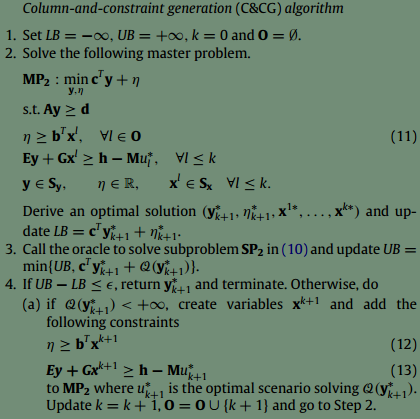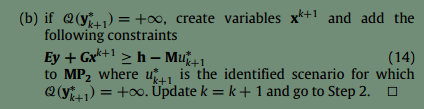# How to add constraints iteratively in CVX?

I want to implement a robust optimization by a column-and-constraint generation framework.
But I’ve no idea how to add a new constraint to a problem in a if-else loop .
Just like the counterpart in YALMIP,which i can deal the constraints as a vector.
if e>0
constraints = [constraints,x>=1]
else
constraints = constraints
end
Could you please show a simple example or pseudo-code of this?
Thank you!
Cheng Kung

The detail of the CC&G algorithm is hereAs long as the if condition determining which constraints to add is on a MATLAB variable and not a CVX variable, then just do the natural thing. Here is a simple example.

``````y=1;
cvx_begin
variable x
minimize(norm(x))
if y >= 0
x >= 1
else
x >= 2
end
cvx_end``````

CVX constraints must be generated during the model generation process—that is, they can’t be stored for later use. So, you’ll have to arrange your code to generate those new constraints from the data, along with all of the original constraints, every time the model is called. Yes, CVX is likely less convenient than YALMIP in this application.